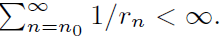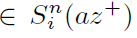##### A generalized Vietoris Mapping

Mirosław Ślosarski

Journal of Advances in Mathematics and Computer Science, Page 89-100
DOI: 10.9734/BJMCS/2015/16103

In this article the properties and applications of generalized Vietoris mappings are presented. The important application of generalized Vietoris mappings is the application to the coincidence theory (see Theorem 4.4).

##### Solution of Heat and Wave – Like Equations by Adomian Decomposition Sumudu Transform Method

Shams E. Ahmed, Tarig M. Elzaki

Journal of Advances in Mathematics and Computer Science, Page 101-111
DOI: 10.9734/BJMCS/2015/9225

In this paper, we use Sumudu transform method and Adomian decomposition method to find solutions of heat and wave – like equations. This method is called the Adomain decomposition Sumudu transform method (ADSTM).

##### Stability Analysis of a Glucose Insulin Dynamic System Using Matrix Lambert W Function

R. Nagarajan, K. Krishnan, C. Monica

Journal of Advances in Mathematics and Computer Science, Page 112-120
DOI: 10.9734/BJMCS/2015/16147

In this paper we investigate the stability of the glucose-insulin dynamical system. We find an explicit expression for the eigenvalues of the dynamical system with single delay. Using matrix function definitions, we use the Lambert W matrix function from which we form the expression.

##### Some New Oscillation Theorems for Second Order Difference Equations with Mixed Neutral Terms

D. Segher, E. Thandapani, S. Pinelas

Journal of Advances in Mathematics and Computer Science, Page 121-133
DOI: 10.9734/BJMCS/2015/16079

Some new sufﬁcient conditions are established for the oscillation of all solutions of the second order
neutral difference equation of the formwhereThe results obtained here are new and further improve and complement
some known results in the literature. Examples are provided to illustrate the main results.

##### Modified Adomian Decomposition Method for Solving Eleventh-order Initial and Boundary Value Problems

Hardik S. Patel, Ramakanta Meher

Journal of Advances in Mathematics and Computer Science, Page 134-146
DOI: 10.9734/BJMCS/2015/16155

A modified Adomian decomposition method is developed for the numerical solution of Eleventhorder initial and boundary value problems. The approximate solutions of the problems are calculated in the form of a rapid convergent series and on one and both grid points. The calculated numerical results show reliability and efficiency of the algorithm given. Two numerical examples of linear and nonlinear problems have been considered to illustrate the efficiency and implementation of the method.

##### A Mathematical Model for Estimating the Weight of Human Beings Using Some Anthropometric Parameters (A Case Study of Taraba State of Nigeria’s Community)

O. D. Ogwumu, S. A. Amoo, F. Y. Eguda, E. O. Adeyefa, S. Abubakar

Journal of Advances in Mathematics and Computer Science, Page 147-158
DOI: 10.9734/BJMCS/2015/10987

The research is concerned with the development of a mathematical model for estimating the body weight of human beings in relation to some of their anthropometric parameters (height and waist sizes). The model was optimized to know whether it is possible for humans to have a maximum or minimum body weight. However, the optimization result showed that there is no specific body weight that could be called a maximum or minimum. Emphasis was laid mainly on a particular proportion of Nigerians from the north- west geopolitical zone (as a case study ) in order to be able to make generalizations about the entire country and beyond. Hence, the population sample for the research was the Taraba State of Nigeria’s Community. Moreover, several recommendations were made at the end of the model analysis which when adhered to, would bring about some medical breakthroughs to the entire human populace.

##### Infinite Matrix Operator, Spline Wavelet Coefficient and Frames

N. A. Sheikh, Devendra Kumar, Mobin Ahmad Syed

Journal of Advances in Mathematics and Computer Science, Page 159-169
DOI: 10.9734/BJMCS/2015/4843

In this paper we study the action of A on f( the spline of order n with nodes az+) and on its wavelet coefﬁcients, where A =is a double inﬁnite matrix operator in a Hilbert space l 2(z+). Also, we make an attempt to ﬁnd frame conditions for A-transform of f.